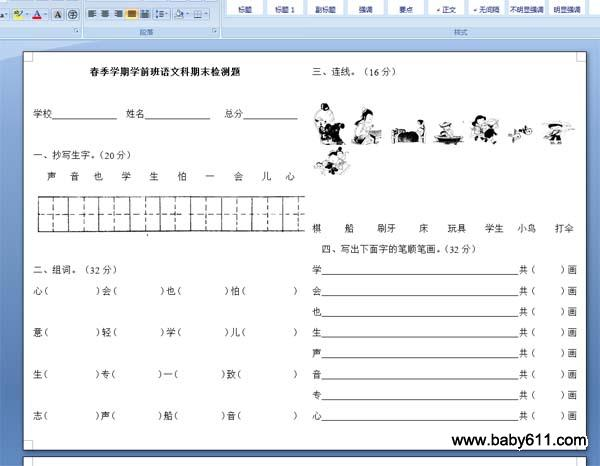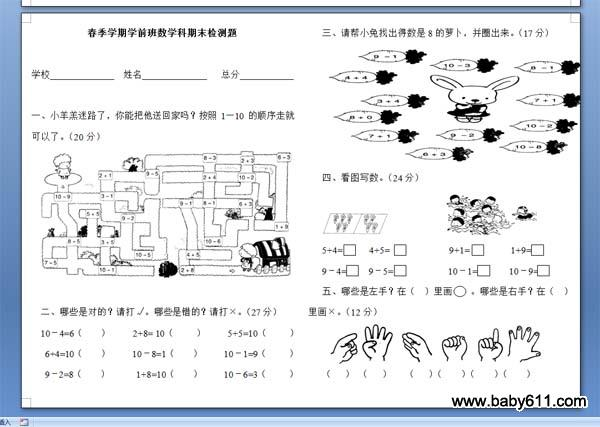春季学期学前班语文、数学期末检测题春季学期学前班语文科期末检测题学校                姓名                 总分一、抄写生字。（20分）声   音   也    学    生    怕    一    会    儿   心二、组词。（32分）心（          ）会（         ）也（         ）怕（          ）意（          ）轻（         ）学（         ）儿（          ）生（          ）专（         ）一（         ）致（           ）志（          ）声（         ）船（         ）音（           ）三、连线。（16分）棋     船     刷牙     床    玩具    学生   小鸟    打伞四、写出下面字的笔顺笔画。（32分）学                                          共（     ）画会                                          共（     ）画也                                          共（     ）画生                                          共（     ）画声                                          共（     ）画音                                          共（     ）画专                                          共（     ）画心                                          共（     ）画春季学期学前班数学科期末检测题学校                姓名                 总分一、小羊羔迷路了，你能把他送回家吗？按照1-10的顺序走就可以了。（20分）二、哪些是对的？请打√。哪些是错的？请打×。（27分）10－4=6（    ）     2+8= 10（     ）     5+5=10（     ）6+4=10（    ）     10－8=1（    ）     10－1=9（     ）9－2=8（    ）      1+8=10（    ）     10－6=3（     ）三、请帮小兔找出得数是8的萝卜，并圈出来。（17分）四、看图写数。（24分）5+4=      4+5=             9+1=         1+9=9－4=     9－5=            10－1=       10－9=五、哪些是左手？在（  ）里画    。哪些是右手？在（  ）里画×。（12分）（   ） （   ）   （   ）（    ）（    ）（    ）

• 幼儿园试卷分类
• 将此试卷分享到：
• 综合试卷最新更新